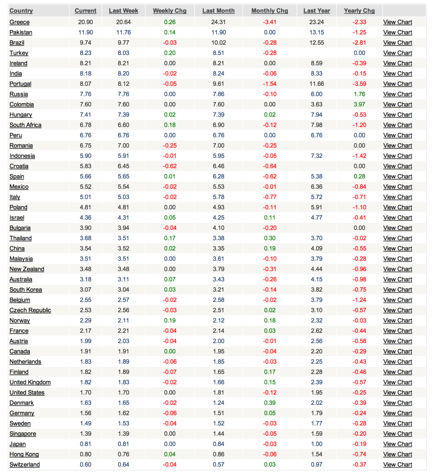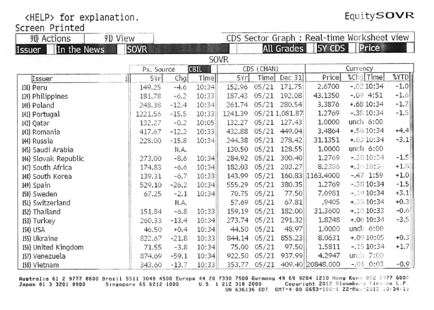Week 2: Estimating a riskfree rate and country risk premium

You are trying to estimate a risk free rate in Peruvian Sol and the country risk premium that you would apply to Peru. You have collected the following information:

Government bond rates (in local currency) by countryCDS spreads by countryIn addition, you have estimated the standard deviation in the Peruvian equity index over the last two years to be 28% and the standard deviation in the Peruvian government bond over the same period at 18%.

The U.S. treasury bond rate is 2%, the historical risk premium of stocks over T.bonds over the last 83 years in the U.S. has been 4.10% and the annualized standard deviation in U.S. equities has been 20%.

1.     Estimate the riskfree rate in Peruvian Sol.

2.     Estimate the total equity risk premium (and the portion of it that is the country risk premium) using three approaches:

a.      Pure bond default spread approach

b.     Relative equity market volatility (Peruvian equity to US equity)

c.      Market volatility adjusted default spread (Peruvian Equity to bond volatility)